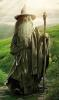Elite indicators :) - page 9029496

timmyhanke:
Can you add Alerts to this indicator , hope it works , looked at the code and it seemed quite short. fx_snipervs_t3_cci.mq4

Timmyhanke, have this one, you get same results with more options, including alerts.

Files:149

my RSI-EMA crosses & filtered idea is here

https://www.mql5.com/en/forum/general

thx....151264

my RSI-EMA crosses & filtered idea is here

https://www.mql5.com/en/forum/general

thx....

Missed it

Will get on it652

Is it possible to program an "adaptive" histogram (MACD type) LinearRegSlope indicator where the histogram shows the difference between the short and long slope?

I think the extern input parameters required are:

int Price = 0

int adaptive = 0 (if 0 then the indicator uses the input shortlength/longlength. if 1 then the indicator uses the short/long cyclemultiplier)

int ShortLength = 20

int LongLength = 35

double CycleAlfa = 0.07

double shortCycleMultiplier = 0.5;

double longCycleMultiplier = 2;

thanks,

jim

Files:149

Missed it Will get on itNo problem.

Question:

TMA bands Centered with sideways signal-line.

Is there such a thing?151264

94315jim:

Is it possible to program an "adaptive" histogram (MACD type) LinearRegSlope indicator where the histogram shows the difference between the short and long slope?

I think the extern input parameters required are:

int Price = 0

int adaptive = 0 (if 0 then the indicator uses the input shortlength/longlength. if 1 then the indicator uses the short/long cyclemultiplier)

int ShortLength = 20

int LongLength = 35

double CycleAlfa = 0.07

double shortCycleMultiplier = 0.5;

double longCycleMultiplier = 2;

thanks,

jim

jim

Try this out :

cycleperiod_v2.mq4

_______________________

PS: it is not a histogram version (yet), but a regular oscillator, but you have now an option to have adaptive or not, and difference or not calculation (so it can do what the previous did - just cleaned up - and can calculate the difference of any combination of two linear regression slopes). let me know if this is enough as is (since it is showing the values very clean) or the histogram version is still required

PPS: Cycle period is needed when the Adaptive option is chosen (otherwise it will not work OK)

Files:
lr_slope.gif 65 kb652

jim Try this out :

cycleperiod_v2.mq4

_______________________

PS: it is not a histogram version (yet), but a regular oscillator, but you have now an option to have adaptive or not, and difference or not calculation (so it can do what the previous did - just cleaned up - and can calculate the difference of any combination of two linear regression slopes). let me know if this is enough as is (since it is showing the values very clean) or the histogram version is still required

PPS: Cycle period is needed when the Adaptive option is chosen (otherwise it will not work OK)

The indicator looks great.

If possible could you add histogram as visually it would be easier to see when the difference is increasing/decreasing.

thank you,

jim271

Please add email/notifications to this indicator when price touches the support or resistance

Thanks

Files:151264

94315jim:

The indicator looks great.

If possible could you add histogram as visually it would be easier to see when the difference is increasing/decreasing.

thank you,

jim

jim

Here is a version with histogram on slope change added : linearregslope_ca_adaptive_histo.mq4

Files:271

Boloti: